# DEGREES Function In ExcelArticle byExcelMojo Team## What Is DEGREES Function In Excel?

The DEGREES function in Excel is an inbuilt Math & Trig function, which converts an angle specified in radians to degrees. Users can use the DEGREES function when they require an angle in degrees while solving trigonometric equations, as Excel uses radians to perform these calculations.

For example, the below table shows a list of angles in radians.

Suppose the requirement is to display the given angles in degrees in column B.

Then, with the given angles in radians and DEGREES function in Excel converting radians to degrees, we can apply the DEGREES() in the target cells to obtain the required data.

In the above example, we input DEGREES function in Excel cell range B2:B6. And each DEGREES function accepts the angle in radians, specified in the corresponding column A cell, to convert the value into degrees.

###### Key Takeaways
• The DEGREES function in Excel converts a value, given in radians, into degrees.
• Users can use the DEGREES function when the requirement is to display an angle in degrees while performing trigonometric equations. The reason is that Excel performs trigonometric operations using angles in radians.
• The DEGREES function accepts one mandatory argument, angle, as input. And the argument value can be a valid negative, positive, zero, integer, decimal, or fractional number.
• The DEGREES function works as per the below formula:
• The PI() returns the Pi-value of 3.141592653589793238.

### Explanation And Uses

A circle’s radius is half the distance across the circle’s center, creating an angle equaling one radian. And one radian equals about 57.3 degrees. The reason is that a complete circle makes 360 degrees or 2 Pi radians.

The following equation represents the above statements.

Or

Furthermore, Excel typically considers angles in radians when the equations to solve are trigonometric.

But for a layman, understanding angle values in degrees is more straightforward. And thus, applying the DEGREES function while performing trigonometric calculations can help obtain angles in degrees instead of radians.

The DEGREES() syntax is as follows:

where,

• angle: The angle specified in radians that you require to convert into degrees equivalent. And it is a mandatory argument.

The uses of the DEGREES Excel function are as follows:

• The DEGREES function is useful while solving trigonometric equations involving Excel functions such as ACOS, ASIN, and ATAN.
• We can use the DEGREES function in mathematical equations when the requirement is to convert a value in radians to degrees.

### How To Use DEGREES Function In Excel?

We can use the DEGREES Excel Function in 2 ways, namely,

1. Access from the Excel ribbon.
2. Enter in the worksheet manually.

#### Method #1 – Access From The Excel Ribbon

First, choose a target cell for output.

Next, select the Formulas tab and click the Math & Trig option drop-down option.

Then, select the DEGREES function, as shown below.

The Function Arguments window appears. Next, enter the argument in the Angle field

Finally, click OK, as shown below.

Likewise, we can use degrees function in excel to obtain results.

#### Method #2 – Enter In The Worksheet Manually

1. Select a target cell for the output.
2. Type =DEGREES( in the cell. [Alternatively, type =D or =DE and double-click the DEGREES function from the Excel suggestions.]
3. Enter the mandatory argument as a cell value or reference and close the brackets. Otherwise, we will face the condition of the DEGREES function in Excel not working.
4. Press Enter to execute and get the required angle in degrees.

The below table contains a set of angles in radians.

Suppose the requirement is to display the given angles in degrees in column C.

As the given angles are in radians and DEGREES function in Excel converts radians to degrees, we can use the DEGREES() in the target cells to obtain the required data.

1. Select the target cell C3 and enter DEGREES().

=DEGREES(B3)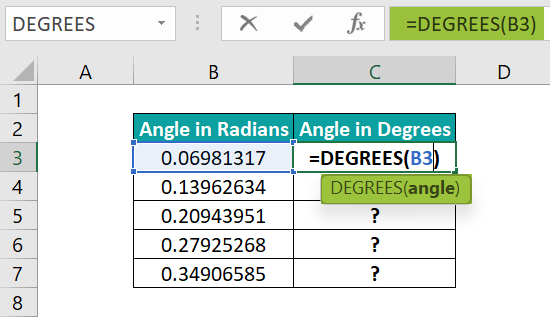2. Press Enter to view the given angle in degrees.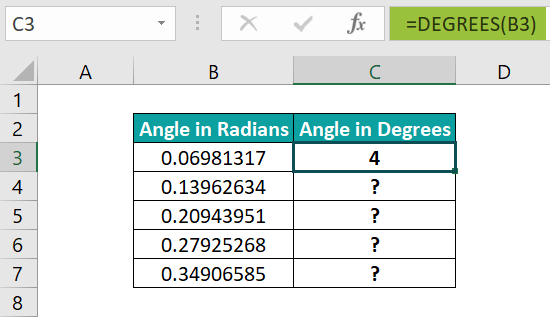Furthermore, entering the following expression and pressing Enter will give the same result.
=DEGREES(0.0698131700797732)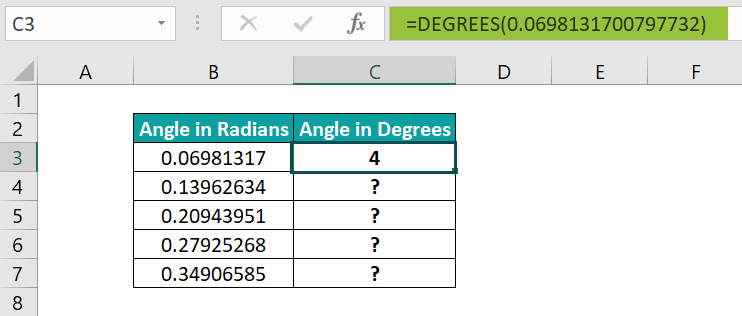[Alternatively, select the target cell C3 and go to FormulasMath & TrigDEGREES to apply the function in the chosen cell.The above step will open the Function Arguments window.
Next, enter the given cell value or reference in the field in the Function Arguments window.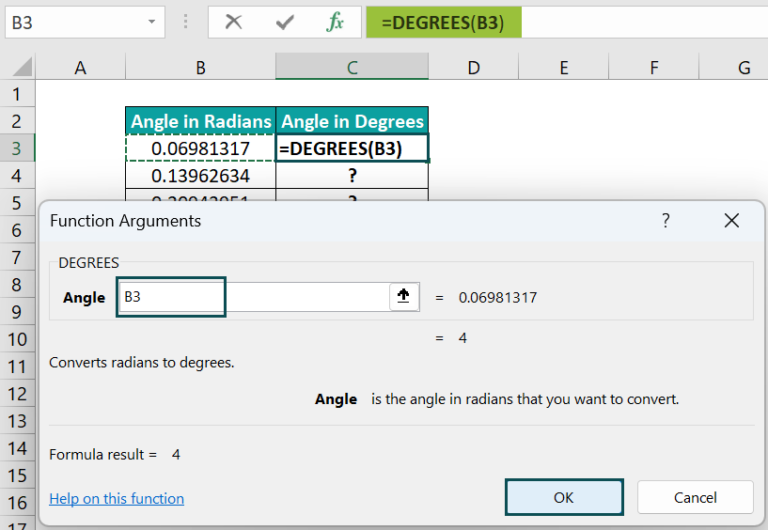Finally, click OK to close the window, and we will see the DEGREES() output in the target cell C3.]

3. Using the fill handle, input DEGREES function in Excel cell range C4:C7.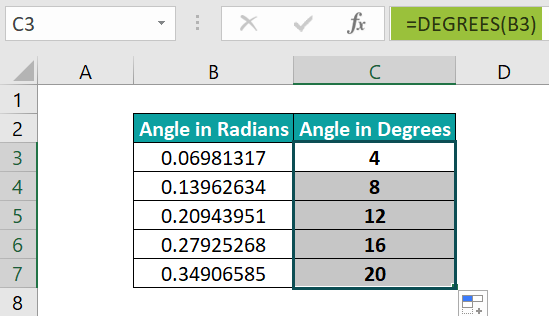Let us see the cell C7 expression to check how the function works. The DEGREES()  accepts the angle value 0.34906585, in radians, as input. And it returns its degrees equivalent, 20°.

### Examples

Check out the below DEGREES() examples to use the function effectively.

#### Example #1

The below table shows a list of negative angles in radians.

Suppose we must display the given negative angles in degrees in column B. Then, we can apply the DEGREES() in the target cells and achieve the required data.

• Step 1: First, select the target cell B2, type DEGREES function in Excel, and then, press Enter.

=DEGREES(A2)

Thus, if the supplied angle to DEGREES() is a negative value in radians, the function returns the negative angle in degrees.

#### Example #2

Let us see how to use the DEGREES() in trigonometric calculations.

For example, the following table contains cosines of different angles.

Suppose we must determine the arccosine or inverse cosine of the specified numbers in column B and display the arccosines in degrees in column C.

Then, applying ACOS() in column B and DEGREES() in column C will help us achieve the required degree values.

• Step 1: To begin with, select cell B3, enter ACOS(), and then, press Enter.

=ACOS(B4)

• Step 2: Next, using the fill handle, update the function in cell range B4:B7.

The ACOS() accepts the given number (the cosine of an angle) as input. And it returns the angle, the inverse cosine, in radians.

• Step 3: Now, select the target cell C3, type DEGREES function in Excel, and then, press Enter.

=DEGREES(C4)

• Step 4: Using the fill handle, implement the DEGREES() in cell range C4:C7.

The DEGREES() accepts the ACOS() output, an angle in radians, as input. And it returns the angle in degrees.

#### Example #3

The following example will help you understand how the DEGREES() operates to ensure we avoid all possibilities of the DEGREES function in Excel not working.

The below table contains a list of angles in radians.

We shall convert the angles specified in radians to degrees using the DEGREES() in column B and the formula mentioned in column C heading in column C.

• Step 1: First, select cell B2, enter DEGREES(), and then, press Enter.

=DEGREES(A2)

• Step 2: Next, use the fill handle to implement the DEGREES() in cell range B3:B6.
• Step 3: Now, select cell C2, enter the below formula, and then, press Enter.

=A2*180/PI()

• Step 4: Using the fill handle, input the formula in cell range C3:C6.

Thus, the DEGREES() output will be the same as when we multiply the given angle in radians by 180 and divide the product by PI() in excel (or 3.14159265358979).

### Important Things To Note

• Ensure we do not supply a non-numeric value as an argument to the DEGREES function in Excel. Otherwise, the function will throw the #VALUE! error in excel.
• For an invalid text as the argument value, the DEGREES function output will be the #NAME? error.

1. Where is the DEGREES function in Excel?

The DEGREES function in Excel is in the Formulas tab. We can select an empty cell and follow the path FormulasMath & TrigDEGREES to apply the function in the chosen cell.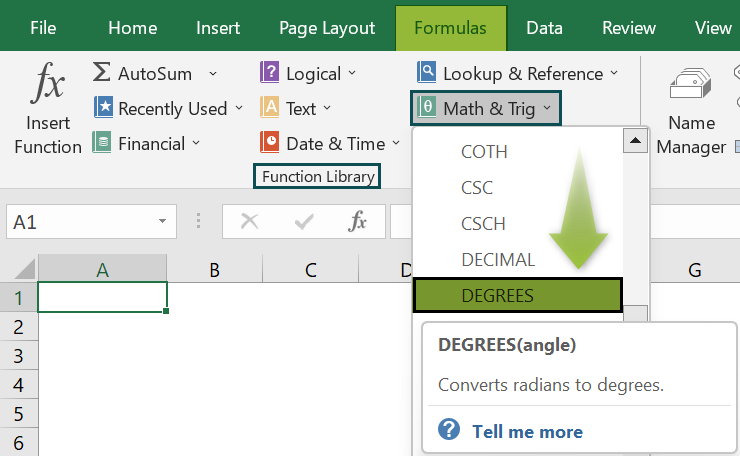2. How to apply DEGREES() in Excel VBA?

We can apply DEGREES() in Excel VBA using the following method:

Application.WorksheetFunction.Degrees(Arg1)

For example, the below table contains a list of angles in radians.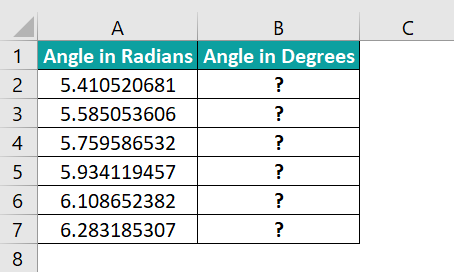Suppose the requirement is to display the given angles in degrees in column B. Then, here is how to use the abovementioned method to apply the DEGREES() in the target cells.

• Step 1: With the active worksheet containing the above table open, access the VBA Editor using Alt + F11.• Step 2: Pick the required VBAProject and choose the Module option under the Insert tab.The Module1 window will open.• Step 3: Enter the VBA code in the Module1 window to apply the DEGREES() in the target cells.

Sub Degrees_fn()
Dim ws As Worksheet
Set ws = Worksheets(“DEGREES_FAQ”)
ws.Range(“B2”) = Application.WorksheetFunction.Degrees(ws.Range(“A2”))
ws.Range(“B3”) = Application.WorksheetFunction.Degrees(ws.Range(“A3”))
ws.Range(“B4”) = Application.WorksheetFunction.Degrees(ws.Range(“A4”))
ws.Range(“B5”) = Application.WorksheetFunction.Degrees(ws.Range(“A5”))
ws.Range(“B6”) = Application.WorksheetFunction.Degrees(ws.Range(“A6”))
ws.Range(“B7”) = Application.WorksheetFunction.Degrees(ws.Range(“A7”))
End Sub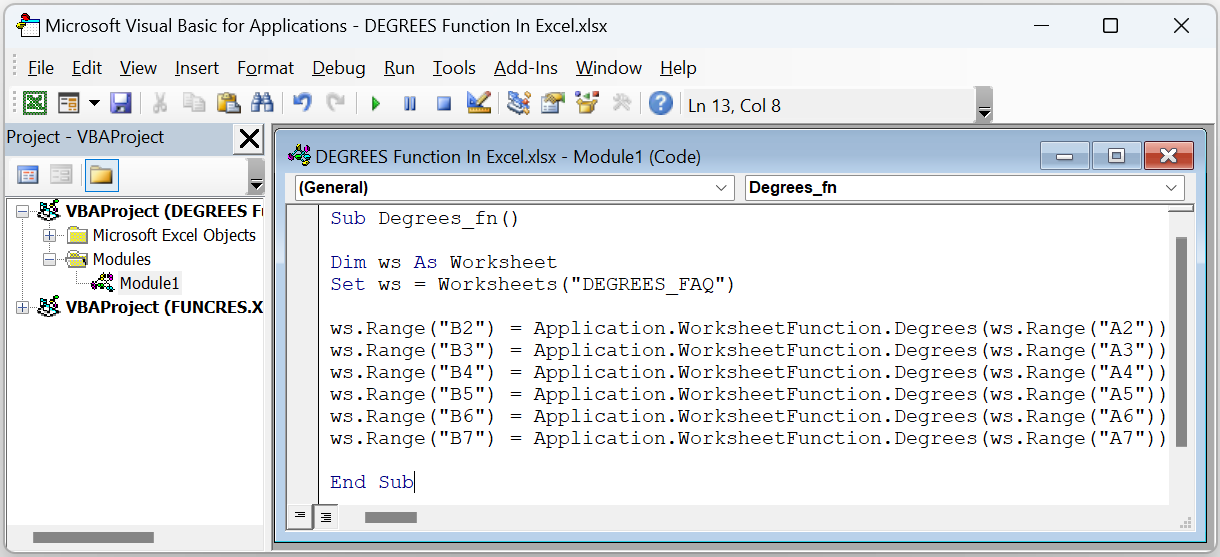• Step 4: Press the run icon to execute the code.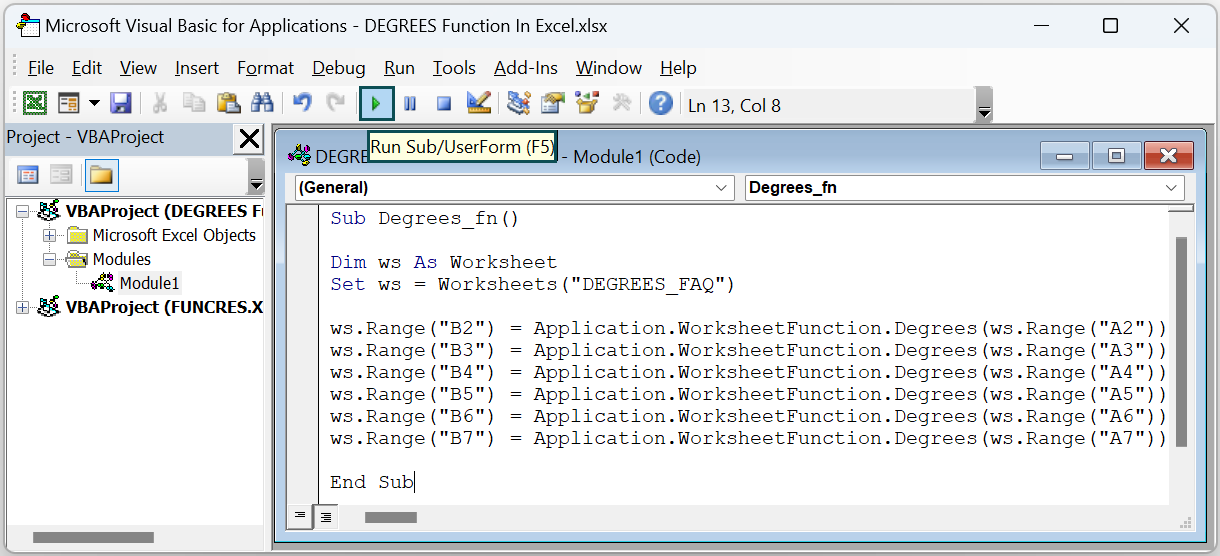Finally, we can open the current sheet to view the required angles in degrees in the target cells.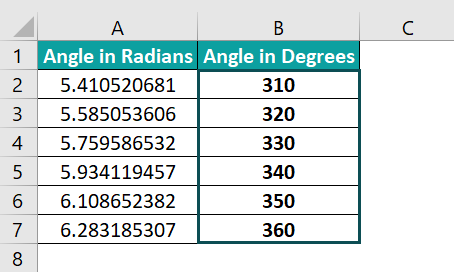3. How to obtain the inverse tangent of a number in degrees in Excel?

We can obtain the inverse tangent of a number in degrees in Excel by using the following formulas:

=DEGREES(ATAN(number))
Or
=ATAN(number)*180/PI()

The ATAN() determines the arctangent or inverse tangent of the specified number,  where the argument number is the tangent of the angle we require.

Thus, the DEGREES() in the first expression converts the ATAN() output, an angle in radians, to degrees.

On the other hand, multiplying the ATAN() output, an angle in radians, by the term 180/PI() gives the angle in degrees.